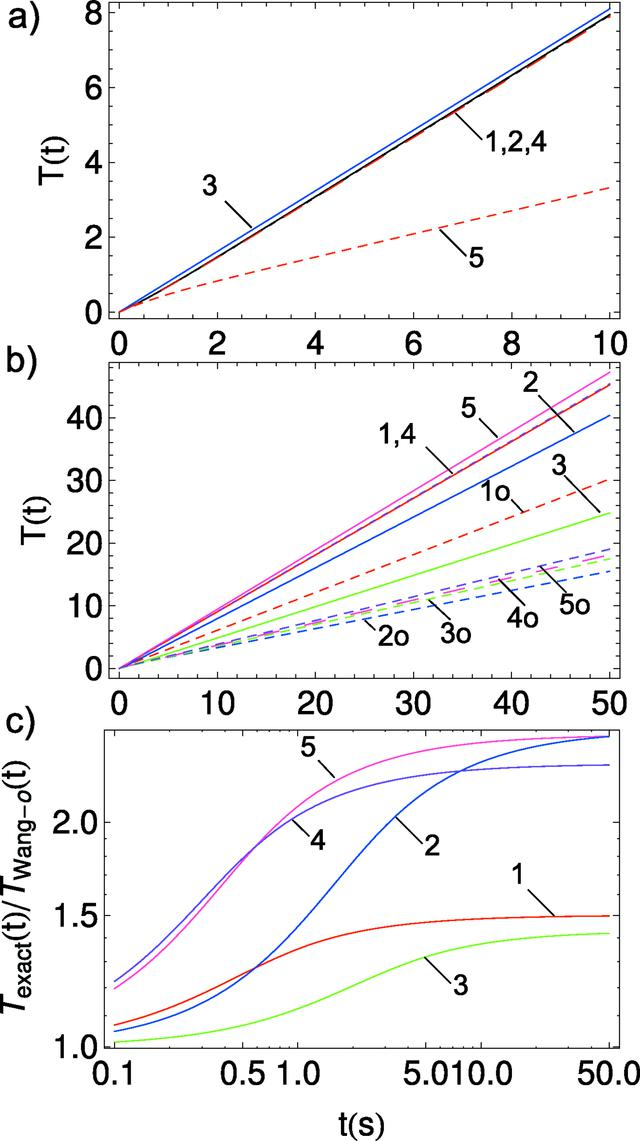disable zoom     view article Figure 12 Comparison of the effective time integral T(t) for all methods applied for A(t) model 1 (exponential). (a) Exact T(t) integration (1, black solid); T(t) approximation (2, black dashed); asymptote of T(t) (3, blue solid); extended Wang method with non-constant sample mass (4, red solid); original Wang method with constant sample mass (5, red dashed). (b) T(t) calculated for all data presented in Fig. 3applying model 1 for A(t). Curve numbers are the same as the symbol numbers in Fig. 3: single numbers (solid curves) are the exact T(t) calculations whereas the numbers with an index `o' (dashed curves) are from the original Wang method. (c) Ratio of the exact T(t) to the Wang original T(t); the curve indexing follows the rule: red curve, (1) is a ratio of the curves 1 and 1o presented in (b), and so on.JOURNAL OFSYNCHROTRONRADIATION
ISSN: 1600-5775
Volume 25| Part 3| May 2018| Pages 833-847